American Journal of Theoretical and Applied Statistics
Volume 4, Issue 6, November 2015, Pages: 480-483

Application of Homotopy Perturbation and Sumudu Transform Method for Solving Burgers Equations

Amjad Ezoo Hamza1, Tarig M. Elzaki1, 2

1Department of Mathematic, Faculty of Sciences, Sudan University of Sciences and Technology, Khartoum, Sudan

2Mathematics Departments, Faculty of Sciences and Arts-Alkamil, University of Jeddah-Saudi, Arabia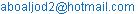(A. E. Hamza)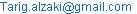(T. M. Elzaki)

Amjad Ezoo Hamza, Tarig M. Elzaki. Application of Homotopy Perturbation and Sumudu Transform Method for Solving Burgers Equations.American Journal of Theoretical and Applied Statistics.Vol.4, No. 6, 2015, pp. 480-483. doi: 10.11648/j.ajtas.20150406.18

Abstract: In this paper, the exact solution of Burgers equations are obtained by using coupling homotopy perturbation and Sumudu transform method (HPSTM), theoretical considerations are discussed, to illustrate the capability and reliability some examples are provided, the results reveal that method is very effective and simple.

Keywords: Burgers Equations, Sumudu Transform, Inverse Sumudu Transform, Homotopy Perturbation Method

Contents

1. Introduction

The Burger's equation  is one of the fundamental equations in fluid mechanics. The burger's equation describes the coupling between diffusion and convection processes.

The standard form of Burgers’ equation is given by: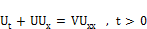(1)

Where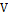is a constant that defines the kinematic viscosity. If the viscosity,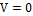, the equation is called In viscid Burgers equation. The in viscid Burgers equation governing gas dynamics. In the viscid Burgers equation has been discussed before as a homogeneous case of the advection problem.

Nonlinear Burger's equation is considered as a simple nonlinear partial differential equations  involving both convection and diffusion in fluid dynamics. Burger introduced this equation  in order to study the interaction of the opposite effects of convection and diffusion in turbulent fluid in a channel. This equation also describes the structure of shock waves, traffic flow and acoustic transmission. A lot of research has been carried out on Burger's equation.

The Cole-Hopf transformation is the commonly used approach. The solution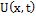was replaced by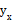in (1) to obtain: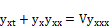(2)

Where by integrating this equation with respect to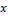we find;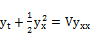(3)

Using the Cole-Hopf transformation;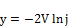(4)

so that:(5)

transforms the nonlinear equation into the heat flow equation;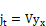(6)

It is clear that nonlinear Burger's equation (1) has been converted to an easily solvable linear equation. This wills results to exact solutions depending on the given conditions. Another technique for deriving solutions to nonlinear Burger's equation in terms of parabolic cylinder functions or Airy functions is the method of symmetry reduction. The symmetry reduction method was applied in an altered way where the Burger's equation transformed to an ordinary differential equation.

2. Homotopy Perturbation Sumudu Transform Method (HPSTM)

Sumudu Transform:

In early 90’s, Watugala  introduced a new integral transform, named the Sumudu transform and applied it to the solution of ordinary differential equation in control engineering problems. The Sumudu transform is defined over the set of functions: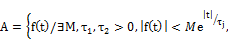by the following formula: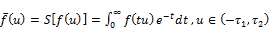(7)

For further detail and properties of this transform, see [2-4, 7-12].

To illustrate the basic idea of this method, Let us consider the Burgers equation of the form:(8)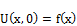.

Taking the Sumudu transform of both sides of eq. (8), subject to the initial condition, we get;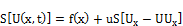(9)

The inverse of Sumudu transform implies that;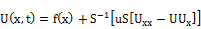(10)

Now, applying the homotopy perturbation method, we get;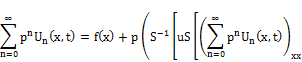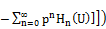(11)

Where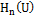are He’s polynomials that represent the nonlinear terms.

The first few components of He’s polynomials, are given by: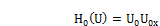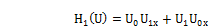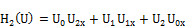(12)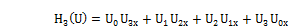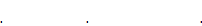Comparing the coefficients of like powers of, we get;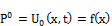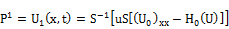(13)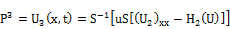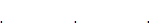Additional components may be computed to increase the accuracy level.

The solution in a series form is as follows. However, the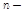term, approximate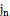can be determined by: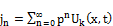(14)

The following examples will illustrate the discussion carried out above by using the homotopy perturbation method.

3. Applications

In this section, we use homotopy perturbation transform method (HPTM) in solving the Burgers equations.

Example 1: Consider the following Burgers equation;(15)

With the initial condition as;.

Taking the Sumudu transform of both sides of eq. (15) subject to the initial condition, we get;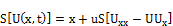(16)

The inverse of Sumudu transform implies that;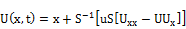(17)

Now, applying the homotopy perturbation method, we get;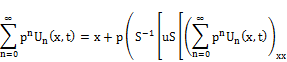(18)

Comparing the coefficients of like powers of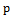, we get;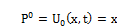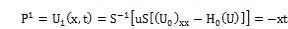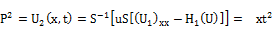(19)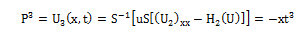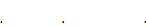And so on. Combining the results obtained for the components, the solution in a series form is given by;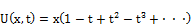(20)

Consequently, the exact solution is given by;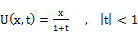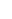(21)

Example 2: let us Consider the following Burgers equation;(22)

With the initial condition as;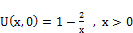.

Taking the Sumudu transform of both sides of eq. (22) subject to the initial condition, we get;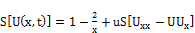(23)

The inverse of Sumudu transform implies that;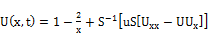(24)

Now, applying the homotopy perturbation method, we get;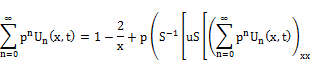(25)

Comparing the coefficients of like powers of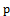, we get;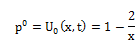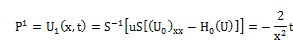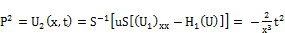(26)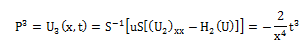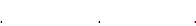And so on. Combining the results obtained for the components, the solution in a series form is given by;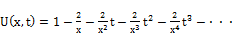(27)

is readily obtained. To determine the exact solution, Eq. (27) can be rewritten as;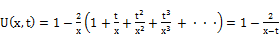(28)

Example 3: Consider the following Burgers equation;(29)

With the initial condition as;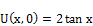.

Taking the Sumudu transform of both sides of eq. (29) subject to the initial condition, we get;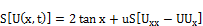(30)

The inverse of Sumudu transform implies that;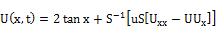(31)

Now, applying the homotopy perturbation method, we get;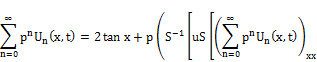(32)

Comparing the coefficients of like powers of, we get;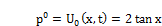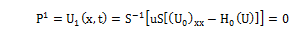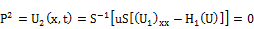(33)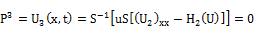Thus, the exact solution is given by;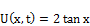(34)

Example 4: Consider the following Burgers equation;(35)

With the initial condition as;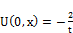,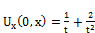.

Applying the Sumudu transform of both sides of eq. (35) subject to the initial condition, we get;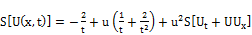(36)

The inverse of Sumudu transform implies that;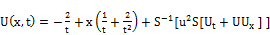(37)

Now, applying the homotopy perturbation method, we get;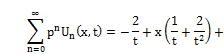(38)

Comparing the coefficients of like powers of, we get;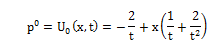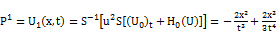(39)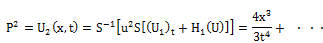And so on. Combining the results obtained for the components, the solution in a series form is given by;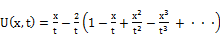(40)

Consequently, the exact solution is given by;(41)

4. Conclusions

For solving, and decomposing nonlinear terms in Burgers equation, one can use Adomian polynomial. The Adomian polynomial is simple, but requires tedious calculation. To overcome this, a new approach using He’s polynomial is proposed for solving the Burgers equation. Called homotopy perturbation and Sumudu transform method (HPSTM). The proposed method reduces computational work compared to classical methods. And, also (HPSTM) may be treated as refinement method to existing numerical techniques, and word finds wide applications.

References

1. G.K. Watugala, Sumudu transform- a new integral transform to solve differential equations and control engineering problems, Math. Engg. Indust., 6 (4) (1998), 319-329.
2. M.A. Asiru, Sumudu transform and the solution of integral equation of convolution type, International Journal of Mathematical Education in Science and Technology, 32 (2001), 906-910.
3. F.B.M. Belgacem, A.A. Karaballi and S.L Kalla, Analytical investigations of the Sumudu transform and applications to integral production equations, Mathematical problems in Engineering, 3 (2003), 103-118.
4. F.B.M. Belgacem and A.A. Karaballi, Sumudu transform fundamental properties investigations and applications, International J. Appl. Math.Stoch. Anal., (2005), 1-23.
5. J.M. Burgers, A mathematical model illustrating the theory of turbulence, Adv. Appl. Mech. 1,171 – 199, (1948).
6. A.M. Wazwaz, Partial Differential Equations: Methods and Applications, Balkema, Leiden,(2002).
7. A. Ghorbani, Beyond adomian’s polynomials: He polynomials, ChaosSolitons Fractals, 39 (2009), 1486–1492.
8. S.T. Mohyud-Din, M.A. Noor and K.I. Noor, Traveling wave solutionsof seventh-order generalized KdV equation using He’s polynomials, International Journal of Nonlinear Sciences and Numerical Simulation, 10(2009), 227–233.
9. J. Biazar, M. Gholami Porshokuhi and B. Ghanbari, Extracting a general iterative method from an adomian decomposition method and comparingit to the variational iteration method, Computers & Mathematics withApplications, 59 (2010), 622–628.
10. S.A. Khuri, A Laplace decomposition algorithm applied to a class of nonlinear differential equations, Journal of Applied Mathematics, 1 (2001),141–155.
11. E. Yusufoglu, Numerical solution of Duffing equation by the Laplacedecomposition algorithm, Applied Mathematics and Computation, 177(2006), 572–580.
12. Y. Khan and F. Austin, Application of the Laplace decomposition method to nonlinear homogeneous and non-homogenous advection equations,Zeitschrift fuer Naturforschung, 65a (2010), 1–5.

 Contents 1. 2. 3. 4.
Article ToolsAbstractPDF(174K)